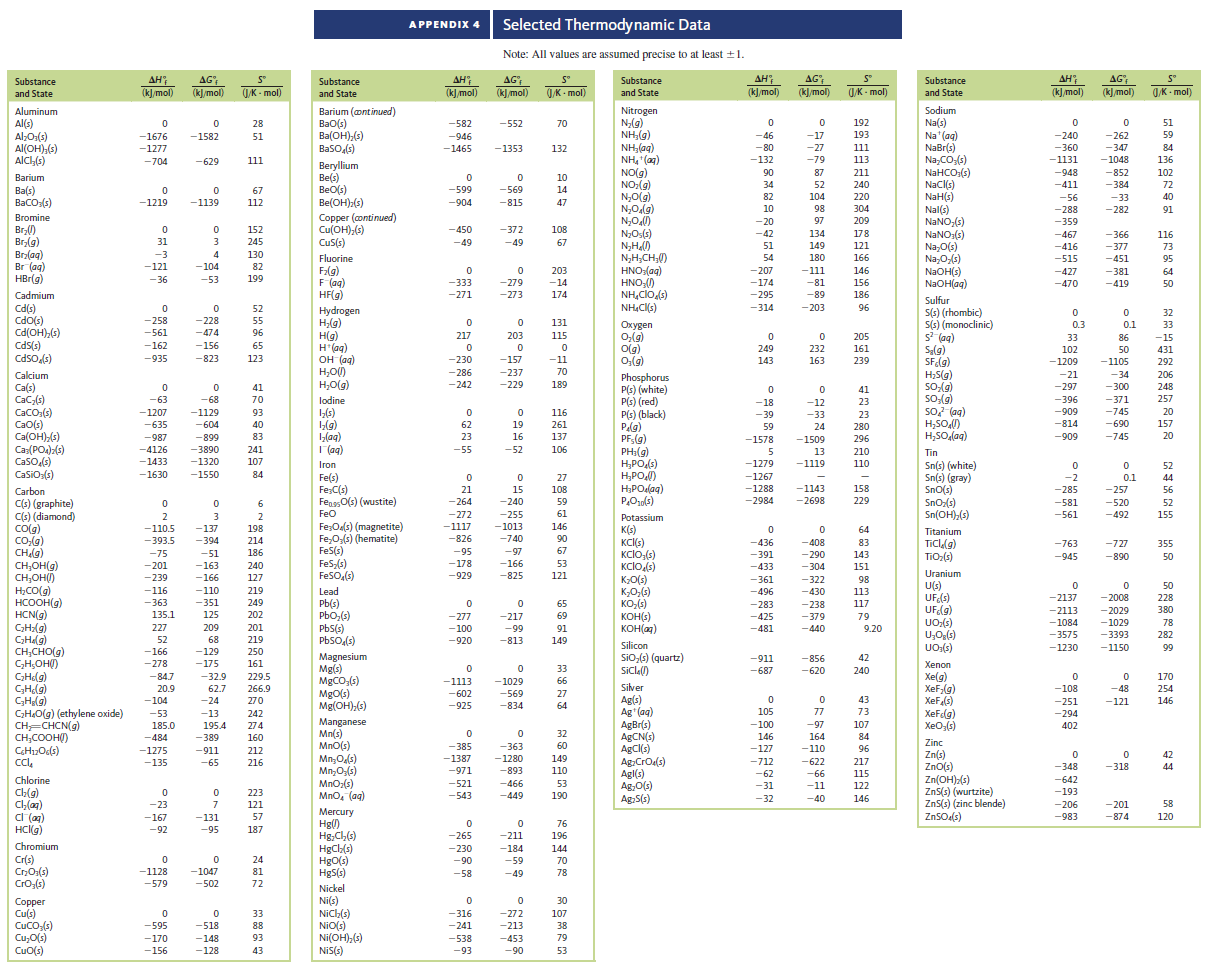# Problem: From data in Appendix 4, calculate ΔH°, ΔS°, and ΔG° for each of the following reactions at 25°C.a. CH4 (g) + 2O2 (g) → CO2 (g) + 2H2O (g)

###### FREE Expert Solution

We’re given the ΔH˚f, ΔG˚f, and ΔS˚f  of each reactant and product:

 ΔH°f (kJ/mol) ΔG°f (kJ/mol) ΔS°f (J/K·mol) CH4(g) – 75.0 – 51.0 186.0 O2(g) 0 0 205.0 CO2(g) – 393.5 – 394.0 214.0 H2O(g) – 242.0 – 229.0 189.0

For ΔH°rxn: We can use the following equation to solve for ΔH˚rxn:

93% (345 ratings)###### Problem Details

From data in Appendix 4, calculate ΔH°, ΔS°, and ΔG° for each of the following reactions at 25°C.

a. CH4 (g) + 2O2 (g) → CO2 (g) + 2H2O (g)